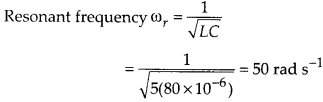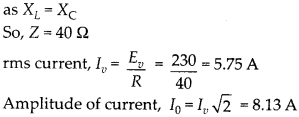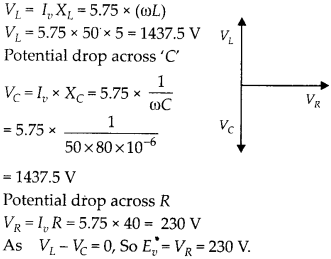Enlightened

# Question 11: NCERT Solutions for 12th Class Physics: Chapter 7-Alternating Current

• 0

Question 11: NCERT Solutions for 12th Class Physics: Chapter 7-Alternating Current

Figure shows a series LCR circuit connected to a variable frequency 230 V source. L = 5.0 H. C = 80 μF, ft = 40 Q(a) Determine the source frequency which drives the circuit in resonance.
(b) Obtain the impedance of the circuit and the amplitude of current at the resonating frequency.
(c) Determine the rms potential drops across -the three elements of the circuit. Show that the potential drop across the LC combination is zero at the resonating

Share

1. Solution:
(a) Condition for resonance is when applied frequency matches with natural frequency.(b) At resonance, impedance Z = R(c) Potential drop across ‘L’Check the complete chapter with solutions.NCERT Solutions for 12th Class Physics: Chapter 7-Alternating Current

• 0